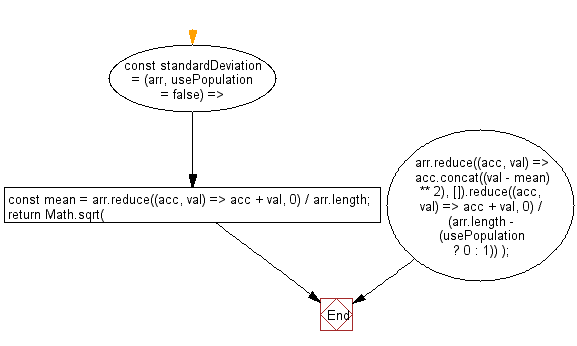# JavaScript: Get the standard deviation of an array of numbers

## JavaScript fundamental (ES6 Syntax): Exercise-225 with Solution

Write a JavaScript program to get the standard deviation of an array of numbers.

• Use Array.prototype.reduce() to calculate the mean, variance and the sum of the variance of the values and determine the standard deviation.
• Omit the second argument, usePopulation, to get the sample standard deviation or set it to true to get the population standard deviation.

Sample Solution:

JavaScript Code:

``````//#Source https://bit.ly/2neWfJ2
const standardDeviation = (arr, usePopulation = false) => {
const mean = arr.reduce((acc, val) => acc + val, 0) / arr.length;
return Math.sqrt(
arr.reduce((acc, val) => acc.concat((val - mean) ** 2), []).reduce((acc, val) => acc + val, 0) /
(arr.length - (usePopulation ? 0 : 1))
);
};

console.log(standardDeviation([10, 2, 38, 23, 38, 23, 21]));
console.log(standardDeviation([10, 2, 38, 23, 38, 23, 21], true));
```
```

Sample Output:

```13.284434142114991
12.29899614287479
```

Flowchart:Live Demo:

See the Pen javascript-basic-exercise-225-1 by w3resource (@w3resource) on CodePen.

Improve this sample solution and post your code through Disqus

What is the difficulty level of this exercise?

Test your Programming skills with w3resource's quiz.

﻿

## JavaScript: Tips of the Day

How to check whether a string contains a substring in JavaScript?

ECMAScript 6 introduced String.prototype.includes:

```const string = "foo";
const substring = "oo";

console.log(string.includes(substring));
```

includes doesn't have Internet Explorer support, though. In ECMAScript 5 or older environments, use String.prototype.indexOf, which returns -1 when a substring cannot be found:

```var string = "foo";
var substring = "oo";

console.log(string.indexOf(substring) !== -1);
```

Ref: https://bit.ly/3fFFgZv